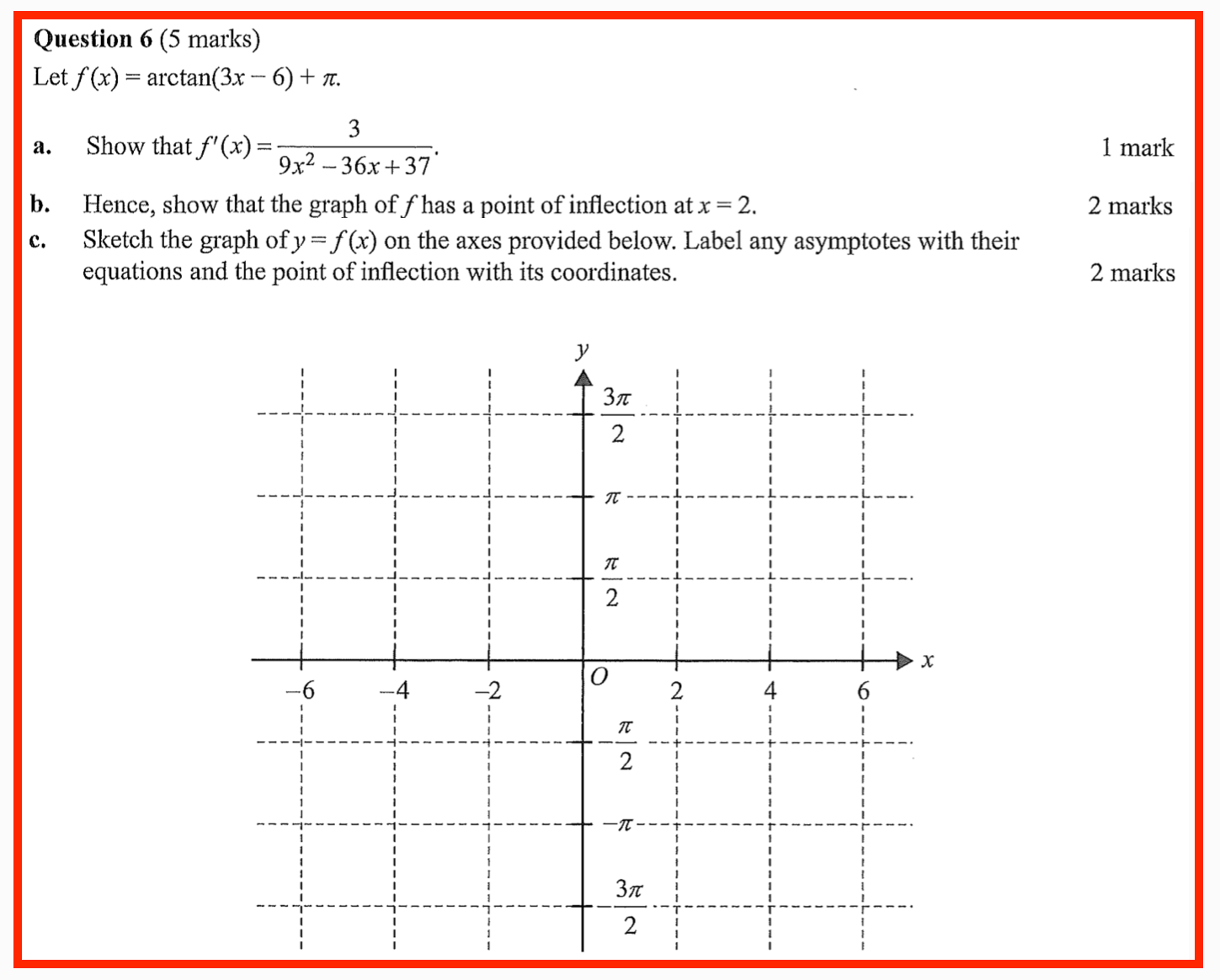# WitCH 47: A Bad Inflection

The question below is from the first 2020 Specialist exam (not online). It has been discussed in the comments here, and the main issues have been noted, but we’ve decided the question is sufficiently flawed to warrant its own post.UPDATE (10/09/21) For those who’d placed a wager, the examination report (Word-doc-VCAA-stupid) indicates that a second derivative argument was expected. Hence, thousands of VCE students no longer have any sense of what VCAA means by “hence”.

## 13 Replies to “WitCH 47: A Bad Inflection”

1.Red Five says:

The word Hence in a VCAA paper always fills me with dread…

There are a few ways this question *could* be answered, many of which have been discussed in the other thread, but clearly VCAA wants the derivative to be used otherwise they wouldn’t use the word HENCE.

Exactly how they want it used is anyone’s guess.

1.John (No) Friend (of VCAA) says:

There are two words here that should create dread.

The first word is “Hence”, for the very reason RF has stated.

The second word is “show”. Who knows what trivial line(s) of superfluous working the tiny minds at VCAA will require.

Put these two words together and you have a multiplying effect of uncertainty. Reading the minds of idiots (with such tiny minds to read in the first place) should not be a prerequisite for getting full marks.

Prove that f has a point of inflection and give its x-coordinate.

1.marty says:

Thanks, John. I don’t see what they can intend expect other than, in effect, what you wrote. But the wording and the lead-up is crazy.

2.marty says:

Yes, the word “Hence” here is incredibly strange.

3.SRK says:

Surely if you’ve at least differentiated the given f'(x) to get f”(x), then you’ve satisfied the “hence” requirement….

1.John (No) Friend (of VCAA) says:

Not necessarily, SRK.

VCAA might require calculation of f”(x) PLUS the statement f”(x) = 0. Or they might want both those things PLUS explicit solution of f”(x) = 0 leading to x = 2.

Potentially three things required for 1 mark.

And then you have to show change in concavity to get the second mark. But maybe VCAA won’t require showing change in concavity for the second mark. In which case, maybe they only want calculation of f”(x) for the first mark and then solution of f”(x) = 0 => x = 2 for the second mark.

Who knows?

And then of course you could argue that f'(x) is the reciprocal of a quadratic whose discriminant is. Therefore f'(x) has a turning point, and the x-coordinate of that turning point is equal to the x-coordinate of the TP of the quadratic. It's trivially equal to 2 by inspection (but how much work does VCAA want to show this?) The conclusion is now obvious.

f''(x) is not required. So where are the two marks now?

As always, students need to keep in mind that they must write a solution that a pinhead could follow.

1.SRK says:

JF that almost sounds like saying that because f’’(x) was not required then it would not be acceptable to use it to answer the question.

I think the explanation for including “hence” is less sinister – VCAA don’t want markers to have to deal with students stuffing up the first derivative on the way to getting the second.

1.marty says:

SRK, I don’t think JF is arguing that using f” is illegal, just that there is a manner of answering the question that doesn’t require it. I don’t think anyone is suggesting that VCAA is being sinister (here), just inept.

2.John Friend says:

SRK, what I’m saying is that f'(x) can be used in two different ways. What will actually be required by VCAA to get those two marks for “Hence”ing and then “show”ing is a complete mystery.

It would have been much better to just ask:

(a) Prove that f has a point of inflection and give its x-coordinate. *3* marks

(b) Sketch the graph …. 2 marks

Students who stuff up the first derivative in (a) can get consequential marks (depending on whether the stuff up trivialises the subsequent calculations). And it should be obvious to any reasonable student thathas a point of inflection where 3x – 6 = 0 => x = 2, so the answer is already known by inspection for my alternate part (a). All that remains is to formally prove it.

Or, if you wanted to test whether students can differentiate an arctan function, then fine, keep part (a) as it is. But don’t have “Hence and “show” in part (b) (and *ahem* hence don’t say that the PI is at x = 2).

Making things easier for PAID assessors who get given SECRET INFORMATION no other teacher is allowed to know should be a much lower priority than asking a straight question and letting students find a straight answer.

2.marty says:

Yes, SRK, one would think calculating f” takes care of the “hence”, but it makes for a damn weird use of “hence”. I guess there’s no rule, but I think of hence as like a lemma-theorem marker; you do the computation (lemma), and then/hence add a little thought to get the interesting conclusion (theorem). But the “hence” here is in no man’s land.

1.Glen says:

Perhaps the author of the question is not a lemma-theorem type of guy.

1.John (No) Friend (of VCAA) says:

There’s no perhaps about it. But I can think of a few things that the author is …

But again, I blame mainly the vettor(s) not the author.

2.marty says:

Well, no, or course they aren’t. But one still expects some consistency in the use of such words. Typically “hence” is both a helpful signpost as well as a command, but here it’s just hugely confusing.

This site uses Akismet to reduce spam. Learn how your comment data is processed.